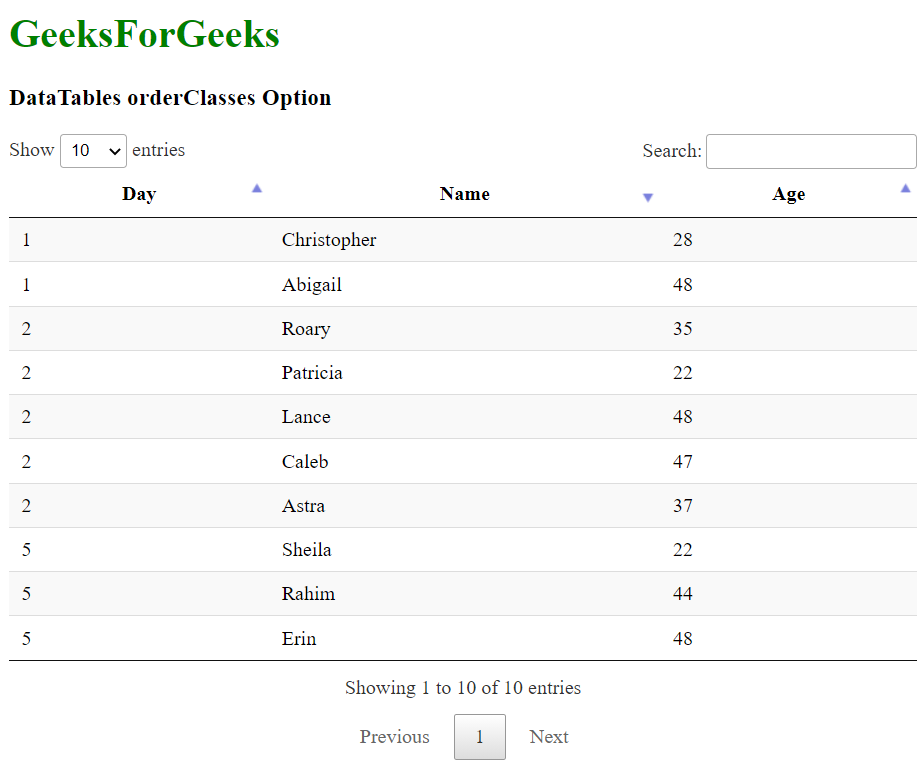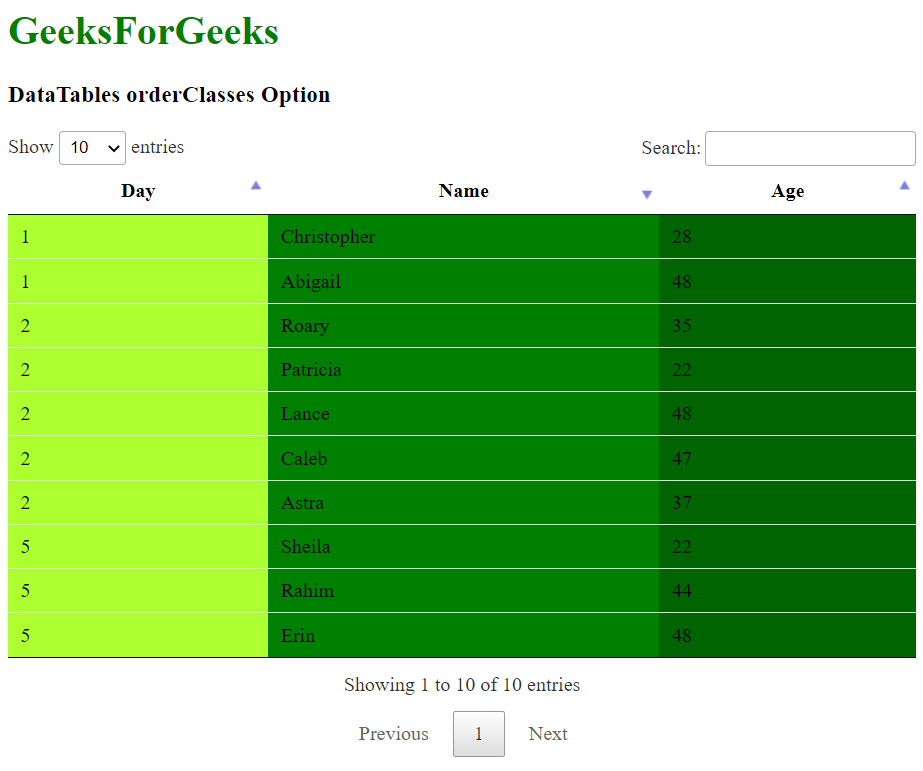# DataTables orderClasses Option

• Last Updated : 22 Jul, 2021

DataTables is jQuery plugin that can be used for adding interactive and advanced controls to HTML tables for the webpage. This also allows the data in the table to be searched, sorted, and filtered according to the needs of the user. The DataTable also exposes a powerful API that can be further used to modify how the data is displayed.

The orderClasses option is used to specify whether the columns that are currently sorted will have a class applied to them or not. By default, DataTables applies the classes sorting_1, sorting_2 and sorting_3 to the columns that are sorted. This adds highlighting to the sorted columns so that they can be distinguished. The number after the class indicates the level of sorting that is used, with the maximum level being 3, after which the last class is repeated.

However, this functionality can be disabled to increase performance on older systems or in cases when a large number of rows have to be manipulated, using this option.

`{ orderClasses: value }`

Parameters: This option has a single value as mentioned above and described below:

• value: This is a boolean value that is used to specify whether currently sorted columns in the table will be highlighted using the predefined classes. The default value is true.

The examples below illustrate the use of this option.

Example 1: In this example, the sorting classes are disabled from being added to the columns. The background color of the three sorting classes has been modified using CSS to make the difference more noticeable.

## HTML

 `<``html``>``<``head``>``  ````  ``<``script` `type``=``"text/javascript"` `          ``src``=``"https://code.jquery.com/jquery-3.5.1.js"``>``  ```` ` `  ````  ``<``link` `rel``=``"stylesheet"``        ``href``=``"https://cdn.datatables.net/1.10.23/css/jquery.dataTables.min.css"``>`` ` `  ````  ``<``script` `src``=``          ``"https://cdn.datatables.net/1.10.23/js/jquery.dataTables.min.js"``>``  ```` ` `  ``<``style``>`` ` `    ``/* Specify the background color in the``    ``classes applied to the sorted columns */``    ``.sorting_1 {``      ``background-color: greenyellow !important;``    ``}`` ` `    ``.sorting_2 {``      ``background-color: green !important;``    ``}`` ` `    ``.sorting_3 {``      ``background-color: darkgreen !important;``    ``}``  ``````<``body``>``  ``<``h1` `style``=``"color: green;"``>``    ``GeeksForGeeks``  ````  ``<``h3``>DataTables orderClasses Option`` ` `  ````  ``<``table` `id``=``"tableID"` `class``=``"display nowrap"``>``    ``<``thead``>``      ``<``tr``>``        ``<``th``>Day``        ``<``th``>Name``        ``<``th``>Age``      ````    ````    ``<``tbody``>``      ``<``tr``>``        ``<``td``>2``        ``<``td``>Patricia``        ``<``td``>22``      ````      ``<``tr``>``        ``<``td``>2``        ``<``td``>Caleb``        ``<``td``>47``      ````      ``<``tr``>``        ``<``td``>1``        ``<``td``>Abigail``        ``<``td``>48``      ````      ``<``tr``>``        ``<``td``>5``        ``<``td``>Rahim``        ``<``td``>44``      ````      ``<``tr``>``        ``<``td``>5``        ``<``td``>Sheila``        ``<``td``>22``      ````      ``<``tr``>``        ``<``td``>2``        ``<``td``>Lance``        ``<``td``>48``      ````      ``<``tr``>``        ``<``td``>5``        ``<``td``>Erin``        ``<``td``>48``      ````      ``<``tr``>``        ``<``td``>1``        ``<``td``>Christopher``        ``<``td``>28``      ````      ``<``tr``>``        ``<``td``>2``        ``<``td``>Roary``        ``<``td``>35``      ````      ``<``tr``>``        ``<``td``>2``        ``<``td``>Astra``        ``<``td``>37``      ````    ````  ````  ``<``script``>`` ` `    ``// Initialize the DataTable``    ``\$(document).ready(function () {``      ``\$('#tableID').DataTable({`` ` `        ``order: [[0, 'asc'], [1, 'desc'], [2, 'asc']],`` ` `        ``// Disable the highlighting of``        ``// columns that are sorted``        ``orderClasses: false,``      ``});``    ``}); ``  ```````

Output:Example 2: In this example, the relevant classes are enabled and added to the columns. The background color of the three sorting classes has been modified using CSS to make the difference more noticeable.

## HTML

 `<``html``>``<``head``>``  ````  ``<``script` `type``=``"text/javascript"` `          ``src``=``"https://code.jquery.com/jquery-3.5.1.js"``>``  ```` ` `  ````  ``<``link` `rel``=``"stylesheet"``        ``href``=``"https://cdn.datatables.net/1.10.23/css/jquery.dataTables.min.css"``>`` ` `  ````  ``<``script` `src``=``          ``"https://cdn.datatables.net/1.10.23/js/jquery.dataTables.min.js"``>``  ```` ` `  ``<``style``>`` ` `    ``/* Specify the background color in the``    ``classes applied to the sorted columns */``    ``.sorting_1 {``      ``background-color: greenyellow !important;``    ``}`` ` `    ``.sorting_2 {``      ``background-color: green !important;``    ``}`` ` `    ``.sorting_3 {``      ``background-color: darkgreen !important;``    ``}``  ``````<``body``>``  ``<``h1` `style``=``"color: green;"``>``    ``GeeksForGeeks``  ````  ``<``h3``>DataTables orderClasses Option`` ` `  ````  ``<``table` `id``=``"tableID"` `class``=``"display nowrap"``>``    ``<``thead``>``      ``<``tr``>``        ``<``th``>Day``        ``<``th``>Name``        ``<``th``>Age``      ````    ````    ``<``tbody``>``      ``<``tr``>``        ``<``td``>2``        ``<``td``>Patricia``        ``<``td``>22``      ````      ``<``tr``>``        ``<``td``>2``        ``<``td``>Caleb``        ``<``td``>47``      ````      ``<``tr``>``        ``<``td``>1``        ``<``td``>Abigail``        ``<``td``>48``      ````      ``<``tr``>``        ``<``td``>5``        ``<``td``>Rahim``        ``<``td``>44``      ````      ``<``tr``>``        ``<``td``>5``        ``<``td``>Sheila``        ``<``td``>22``      ````      ``<``tr``>``        ``<``td``>2``        ``<``td``>Lance``        ``<``td``>48``      ````      ``<``tr``>``        ``<``td``>5``        ``<``td``>Erin``        ``<``td``>48``      ````      ``<``tr``>``        ``<``td``>1``        ``<``td``>Christopher``        ``<``td``>28``      ````      ``<``tr``>``        ``<``td``>2``        ``<``td``>Roary``        ``<``td``>35``      ````      ``<``tr``>``        ``<``td``>2``        ``<``td``>Astra``        ``<``td``>37``      ````    ````  ````  ``<``script``>`` ` `    ``// Initialize the DataTable``    ``\$(document).ready(function () {``      ``\$('#tableID').DataTable({`` ` `        ``order: [[0, 'asc'], [1, 'desc'], [2, 'asc']],`` ` `        ``// Enable the highlighting of``        ``// columns that are sorted``        ``orderClasses: true,``      ``});``    ``}); ``  ```````

Output:My Personal Notes arrow_drop_up